NCERT Solutions for Class 7 Maths Chapter 2 Fractions and Decimals Ex 2.3 are part of NCERT Solutions for Class 7 Maths. Here we have given NCERT Solutions for Class 7 Maths Chapter 2 Fractions and Decimals Ex 2.3.

 Board CBSE Textbook NCERT Class Class 7 Subject Maths Chapter Chapter 2 Chapter Name Fractions and Decimals Exercise Ex 2.3 Number of Questions Solved 8 Category NCERT Solutions

## NCERT Solutions for Class 7 Maths Chapter 2 Fractions and Decimals Ex 2.3

Question 1.
Find: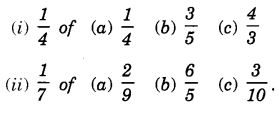Solution: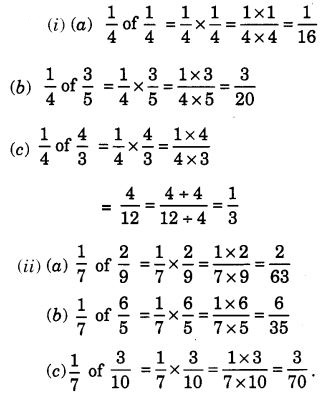Question 2.
Multiply and reduce to lowest form (if possible):Solution: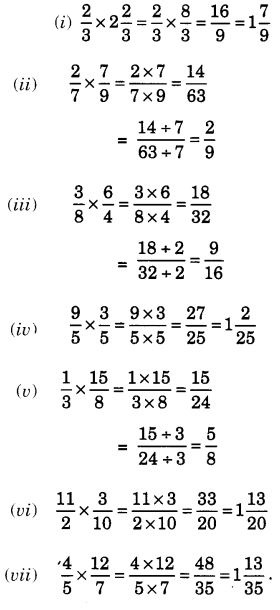Question 3.
Multiply the following fractions: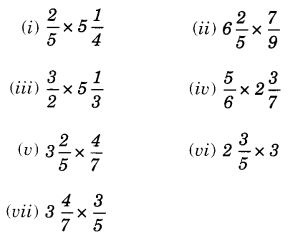Solution: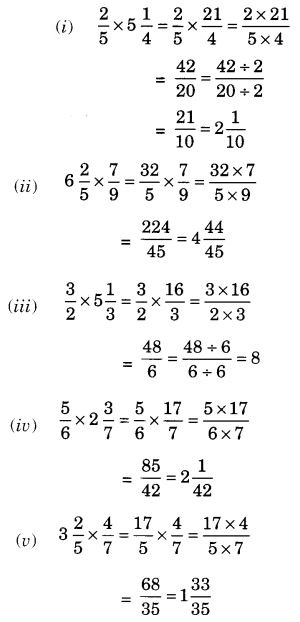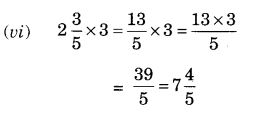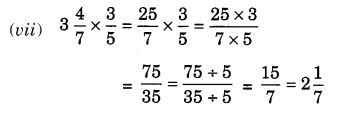Question 4.
Which is greater: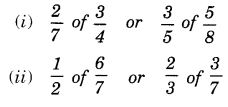Solution: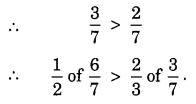Question 5.
Saili plants 4 saplings, in a row, in her garden. The distance between two adjacent saplings is $$\frac { 3 }{ 4 }$$ m. Find the distance between the first and the last sapling.
Solution: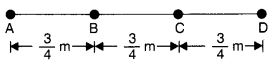Let A, B, C and D be the four saplings planted in a row.
Distance between two adjacent saplings = $$\frac { 3 }{ 4 }$$ m
∴ Distance between the first and the last sapling = AD = 3 × AB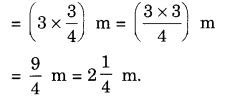Question 6.
Lipika reads a book for 1 $$\frac { 3 }{ 4 }$$ hours everyday. She reads the entire book in 6 days. How many hours in all were required by her to read the book?
Solution:
Hours in all required by Lipika to read the book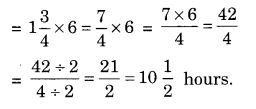Question 7.
A car runs 16 km using 1 litre of petrol. How much distance will it cover using 2 $$\frac { 3 }{ 4 }$$ litres of petrol?
Solution:
Distance covered by the car using 2 $$\frac { 3 }{ 4 }$$ litres of petrol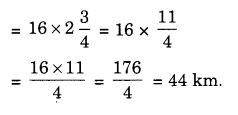Question 8.Solution: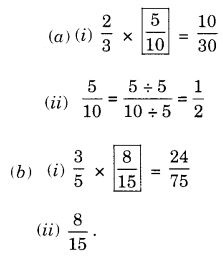We hope the NCERT Solutions for Class 7 Maths Chapter 2 Fractions and Decimals Ex 2.3 help you. If you have any query regarding NCERT Solutions for Class 7 Maths Chapter 2 Fractions and Decimals Ex 2.3, drop a comment below and we will get back to you at the earliest.